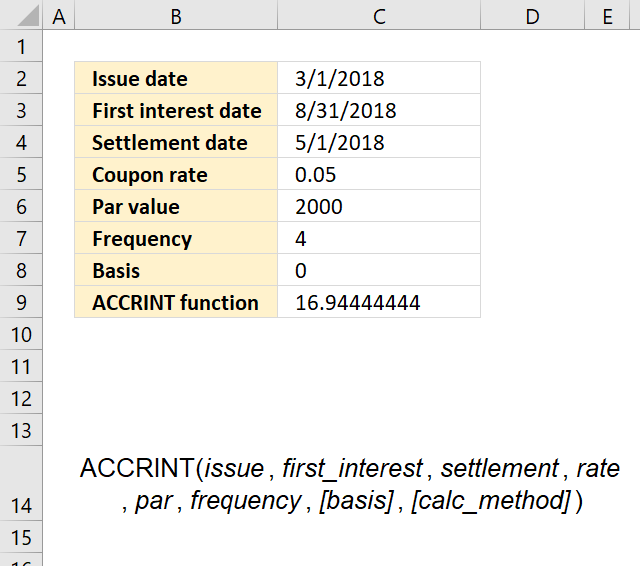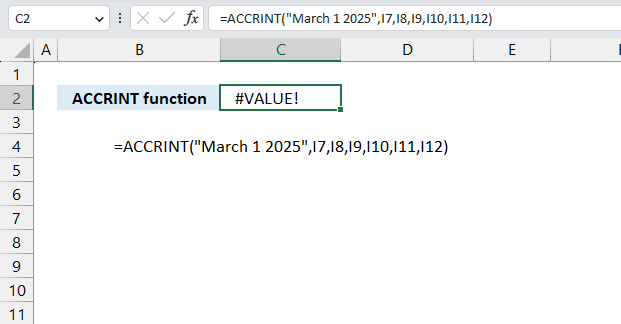Author: Oscar Cronquist Article last updated on May 08, 2023What is the ACCRINT function?

The ACCRINT function calculates the accrued interest for a security that pays periodic interest.

What is a security?

A security that pays interest is a bond that investors use to loan money to a company or a government in exchange for interest payments. The interest rate is fixed and does not change over the life of the security.

What is accrued interest?

In finance, accrued interest is the interest on a bond that has accumulated since the last coupon payment.

What is periodic interest?

The interest payments are usually made periodically, such as every three, six months or twelve months, until the security matures and the principal amount is repaid.

## 1. ACCRINT Function Syntax

ACCRINT(issue, first_interest, settlement, rate, par, frequency, [basis], [calc_method])

## 2. ACCRINT Function Arguments

 issue Required. The security's issue date. first_interest Required. The security's first interest date. settlement Required. The security's settlement date. The security settlement date is the date after the issue date when the security is traded to the buyer. rate Required. The security's annual coupon rate. par Required. The security's par value. Default value is \$1,000. frequency Required. The number of coupon payments per year.
frequency payments
1 Annual
2 Semiannual
4 Quarterly
 [basis] Optional. The type of day count basis to use.
Basis Day count basis
0 (default value) US (NASD) 30/360
1 Actual/actual
2 Actual/360
3 Actual/365
4 European 30/360
 [calc_method] Optional. A logical value that specifies the way to calculate the total accrued interest when the date of settlement is later than the date of first_interest.
calc_method Function returns
TRUE Returns the total accrued interest from issue to settlement.
FALSE Returns the accrued interest from first_interest to settlement. Default value.

## 3. ACCRINT function ExampleThe image above shows the following details for a bond:

Issue date: March 1, 2018
First interest date: August 31, 2018
Settlement date: May 1, 2018
Coupon rate: 5% (0.05)
Par value: \$2,000
Frequency: 4 (quarterly payments)
Basis: 0 (US (NASD) 30/360)

Formula in cell C9:

=ACCRINT(C2,C3,C4,C5,C6,C7,C8)

The ACCRINT function requires Excel dates to work properly, the image above shows Excel dates in cells C2, C3, and C4. The result is 16.9444444444444.

## 4. ACCRINT function #VALUE! errorThe ACCRINT function may return a #VALUE! error if dates are entered as text values, the image above shows the #VALUE! error in cell C2.

Use the DATE function to enter dates (hard coded dates) in the ACCRINT function . For example, DATE(2018,3,1). The DATE function converts the specified year, month, and day to an Excel date (number).

The following formula shows how to use hard coded values in the ACCRINT function:

=ACCRINT(DATE(2018,3,1),DATE(2018,8,31),DATE(2018,5,1),0.05,2000,4,0)

The ACCRINT function returns the #NUM! error value:

• if the rate is less than 0 (zero).
• if par value is less than 0 (zero).
• if the frequency is not 1,2, or 4.
• basis is less than 0 (zero) or larger than 4.
• issue date is equal or larger than settlement date.

What is hard coded values in a formula?

Hard coded values in Excel are values that are entered directly into a formula instead of using cell references.

## 5. Get Excel file

### Get the Excel fileHow-to-use-the-ACCRINT-functionv2.xlsx